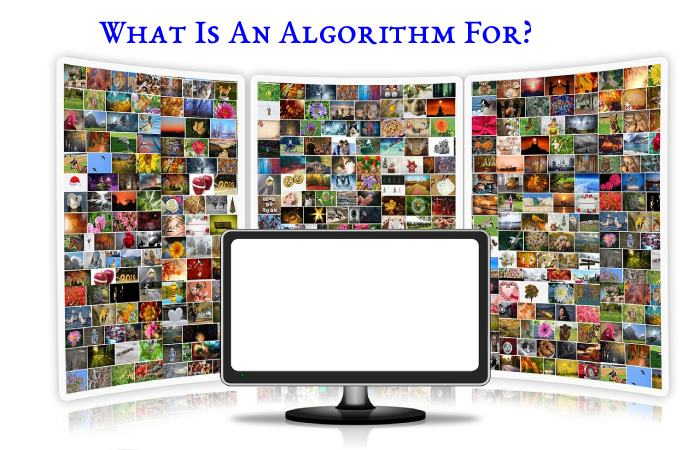## Introduction

An algorithm is a sequence of sequential instructions, thanks to which specific processes can be carried out and given a response to particular needs or decisions. These are ordered and finite sets of steps that allow us to solve a problem or make a decision.

Algorithms have nothing to do with programming languages since the same algorithm or flowchart can be represented in different programming languages

Algorithms are also prevalent in mathematics and logic and are the basis for manufacturing user manuals, instruction booklets, etc. Its name comes from the Latin algorithms, and this last name comes from the Persian mathematician Al-Juarismi. One of the best-known algorithms in mathematics is the one attributed to Euclid, to obtain the greatest common divisor of two positive integers, or the so-called “Gauss method” to solve systems of linear equations.

## Characteristics Of The Algorithms

### Computer Algorithm

An algorithm must offer a result based on its functions.

The algorithms have the following characteristics:

### Sequential

The algorithms operate in sequence. Therefore, they must be process one at a time.

Accurate. Algorithms must be precise in approach to the subject. That is, they cannot be ambiguous or subjective.

### Organized

The algorithms must set in the precise sequence for their reading to make sense and solve the problem.

### Finite

Every sequence of algorithms must have a specific purpose. Therefore, it cannot be prolong to infinity.

### Concrete

Every algorithm must offer a result based on the functions it fulfills.

The same algorithm before input elements must always give the same results.

## What Is An Algorithm For?### Put It Very Simply

An algorithm is use to solve a problem step by step. It is a series of ordered and sequenced instructions to guide a specific process.

In Computer Science, however, algorithms constitute the skeleton of the processes that will later be codify and programmed to be carry out by the computer.

## Types Of Algorithms

There are four types of algorithms in computer science:

### Computational Algorithms

An algorithm whose resolution depends on computation and which can be performed by a calculator or computer without difficulty.

### Non-Computational Algorithms

Those that do not require the processes of a computer to solve or whose steps are exclusive for the resolution by a human being.

### Qualitative Algorithms

It is an algorithm whose resolution involves not numerical calculations but logical and formal sequences.

### Quantitative Algorithms

On the contrary, it is an algorithm that depends on mathematical calculations to find its resolution.

## Parts Of An Algorithm

• Every algorithm must consist of the following parts:
• Input or input. The information of the data that the needs to operate.
• This is the formal logical operation that the algorithm will undertake with what is receive from the input.
• Output or exit. The results obtained from the process on the input, once the execution of the algorithm has finished

## Conclusion

These algorithms do not find the optimal global solution since, when making a decision, they only consider the information of the decisions they have made so far and not the future ones they may adopt. However, some cases in which greedy algorithms reach optimal solutions are: